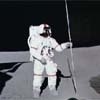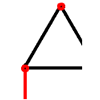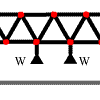#### You may also like### Lunar Leaper

Gravity on the Moon is about 1/6th that on the Earth. A pole-vaulter 2 metres tall can clear a 5 metres pole on the Earth. How high a pole could he clear on the Moon?### Bridge Builder

In this short problem we investigate the tensions and compressions in a framework made from springs and ropes.### More Bridge Building

Which parts of these framework bridges are in tension and which parts are in compression?

# Wobbler

##### Age 16 to 18Challenge Level
This solution was submitted by Andrei from Tudor Vianu National College, Bucharest, Romania.

To determine the position of the centre of gravity of the body, knowing the position of the centre of gravity for the individual bodies (i.e. the hemisphere and the circular cone), I have firstly to determine the masses of the bodies, assuming both have the same density, $\rho$.

The total mass is: $${2\over 3}\rho\pi a^3 + {1\over 3}\rho \pi a^2h.$$ I use the positions of their centres of gravity for the two distinct bodies from the hint. Taking moments about the centre of gravity of the hemisphere I obtain: $$(m_1+m_2)d = m_2\left({h\over 4} + {3a\over 8}\right)$$ where the distance $d$ is measured from the centre of gravity of the hemisphere. This gives $$\bar{x}= h + {3a^2-h^2\over 4(h+2a)}$$ from the vertex of the cone.

Label the vertex of the cone as $V$, the centre of this circular face (on the axis of symmetry) as $O$ and the point where the axis of symmetry cuts the curved surface of the hemisphere as $B$.

(i) If $h = a$, then the centre of gravity is situated in the interior of the hemisphere at a distance $a/6$ from the centre of the circular base. For this position of the centroid (and all points inside the hemisphere) the body rolls to rest with the lowest point of the hemisphere $B$ in contact with the table. The equilibrium corresponding to this position is stable, because if the body is displaced from this position, the torque of the gravitational force in respect to the point of contact with the horizontal plane, which is the point in respect to which the body could rotate, determines the body to come back to its initial position.

(ii) For $h=2a$ the centre of mass of the solid is at a distance $a/16$ from $O$ inside the cone. For this position of the centre of mass (and all positions inside the cone) the body will roll to a position of equilibrium with a generator of the cone in contact with the table. The only exceptions are when it is placed on $V$ or $B$ with the axis of symmetry vertical which are unstable positions of equilibrium.

(iii)If the body remains in equilibrium with any point of the hemisphere in contact with the plane then the centre of gravity must be at $O$ so that the centre of gravityis always vertically above the point of contact. This gives $h=a\sqrt 3$. In this vase the cross section of the cone by a plane of symmetry will be an equilateral triangle, so the angle of the cone will be $60^o$. The equilibrium is unstable when the point of contact is on the rim between the hemisphere and the cone, and stable for all other points of contact between the hemisphere and the table.

(iv) In summary
• for $h< \sqrt 3$ the body comes to rest with $B$ in contact with the table and equilibrium is stable.
• for $h= \sqrt 3$ the body will rest in stable equilibrium if it is placed on the table either with a point of the hemisphere in contact with the table or with a generator of the cone in contact with the table. If it is placed with a point on the rim between the cone and the hemisphere in contact with the table then the equilibrium is semi-stable; if tipped towards the cone it will fall to rest on a generator, if tipped the other way it will rest wherever it is moved to with the hemisphere in contact with the table.
• for $h> \sqrt 3$ the body will roll to a stable equilibrium position with a generator in contact with the table. The exceptional cases are unstable equilibrium with $V$ or $B$ in contact with the table.